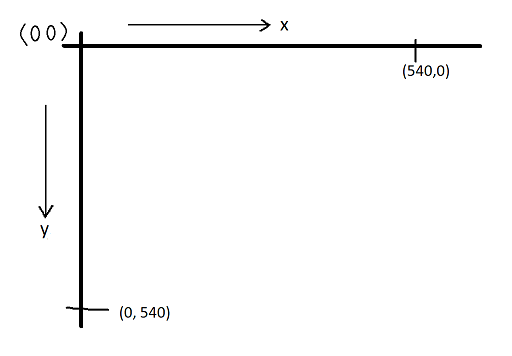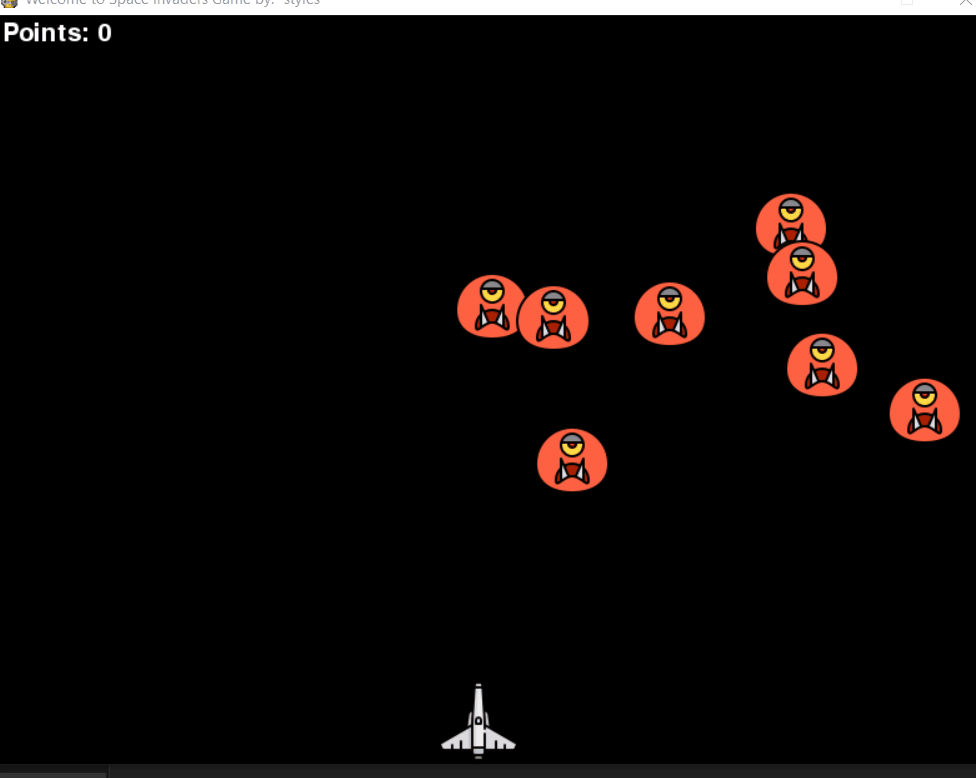Building Space Invaders Using PyGame – Python

• Last Updated : 22 Nov, 2021

Approach:

• Import the required module.
• Initialize the pygame.
• Create three functions:
• isCollision(): Which tells us whether the collision has occurred or not?
• game_over(): Which returns True or False on the basis of which the code decided if the game has ended.
• show_score(x, y): This shows the score on the screen
• Create an infinite loop to execute the code continuously.

isCollision():

It’s very simple actually. Before explaining this, we want you to take a look at the collision portion of the code inside the game loop first below:

Python3

 # Collisioncollision = isCollision(bullet_X, invader_X[i], bullet_Y, invader_Y[i])if collision:        score_val += 1        bullet_Y = 600        bullet_state = "rest"        invader_X[i] = random.randint(64, 736)        invader_Y[i] = random.randint(30, 200)        invader_Xchange[i] *= -1

The value returned by the “isCollision()” function is being stored inside the “collision” variable. The value returned by the function is either True or False based on the criteria set for collision inside the function. Let’s take a look at what is inside the isCollision() function:

Python3

 def isCollision(x1, x2, y1, y2):    distance = math.sqrt((math.pow(x1 - x2, 2)) + (math.pow(y1 - y2, 2)))    if distance <= 50:        return True    else:        return False

The criteria for collision set inside the function is the simplest thing as the distance between the bullet and the invader (our enemy). As you can see the formula used for calculating distance is something that every student study in their high school mathematics class. It’s the formula of the distance between two points having coordinates (x1, y1) and (x2, y2) which are being passed as parameters of the isCollision() function.

Here, we have set the criteria that if the value of the distance is less than or equal to 50, then it means collision has occurred. The value is chosen on the basis of the height and width of the png image used for the bullet and the invader. The value can be tweaked as per your own requirements by using the trial and error method.

So, whenever the position of the bullet and the invader changes then the isCollision() function checks if a collision has occurred or not. That is the reason why it is being called inside the game loop.

game_over():

Which returns True or False on the basis of which the code decided if the game has ended. For understanding the game_over() function, let’s take a look at the below snippet of code which is present inside the game loop:

Python3

Before getting into the explanation of code, it is recommended to know about the coordinate system followed in pygame. Take a look at the image below:So, the criteria for game over is also collision. When the y-coordinate of the invader is greater than the spaceship i.e., 450 (y-coordinate of the spaceship), and the distance between the invader and the spaceship is less than 80 then a collision occurs and the game_over() function is called followed by the explosion sound.

The following lines will make it more clear:

Python3

 # y-coordinate of invader more than 450if invader_Y[i] >= 450:            # distance between the x-coordinate of invader        # and spaceship        if abs(player_X-invader_X[i]) < 80:            #....... rest code .....#

The above is checked for all the invaders present in the game which is ensured by a for-loop at the starting.

show_score(x, y):

It shows the score on the screen.

Python3

 def show_score(x, y):    score = font.render("Points: " + str(score_val),                        True, (255, 255, 255))    screen.blit(score, (x, y))

The only thing show_score() function is doing is showing the score on the screen in a proper font selected by the user.

Every time a collision between the bullet and the invaders is happening a variable “score_val” is being incremented. This variable is then being displayed on the screen by the show_score() function as can be seen in the above code snippet.

Python3